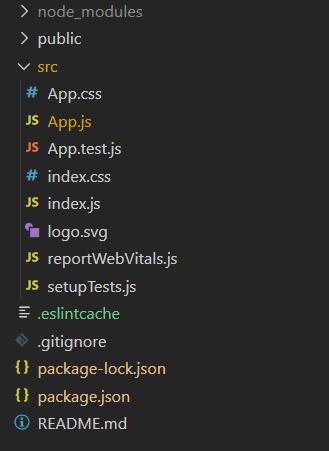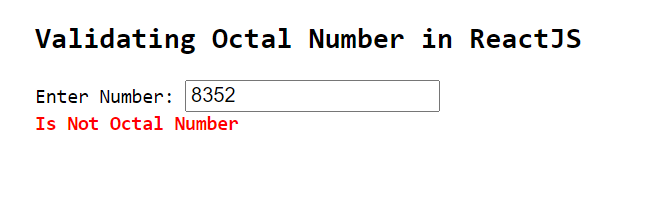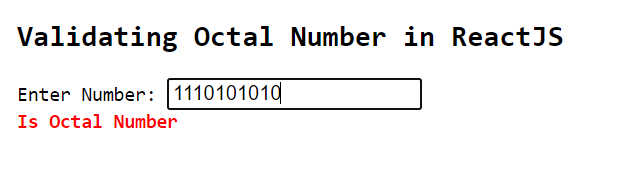# How to validate Octal number in ReactJS?

• Last Updated : 31 Dec, 2020

The Octal numeral system is the base-8 number system which uses the digits 0 to 7. It is also known as Oct for short. The following example shows how to validate the user entered data and check whether it is valid or not using the npm module in the ReactJS application.

Creating React Application And Installing Module:

Step 1: Create a React application using the following command:

`npx create-react-app foldername`

Step 2: After creating your project folder i.e. foldername, move to it using the following command:

`cd foldername`

Step 3: After creating the ReactJS application, Install the validator module using the following command:

`npm install validator`

Project Structure: It will look like the following.Project Structure

App.js: Now write down the following code in the App.js file. Here, App is our default component where we have written our code.

## Javascript

 `import React, { useState } from ``"react"``;``import validator from ``'validator'`` ` `const App = () => {`` ` `  ``const [errorMessage, setErrorMessage] = useState(``''``)``   ` `  ``const validate = (value) => {``   ` `    ``if` `(validator.isOctal(value)) {``      ``setErrorMessage(``'Is Octal Number'``)``    ``} ``else` `{``      ``setErrorMessage(``'Is Not Octal Number'``)``    ``}``  ``}`` ` `  ``return` `(``    ``
``      ``
``        ``

Validating Octal Number ``in` `ReactJS

``        ``Enter Number: validate(e.target.value)}>
``        ``{errorMessage}``      ``
``    ``
``  ``);``}`` ` `export ``default` `App`

Step to Run Application: Run the application using the following command from the root directory of the project:

`npm start`

Output:

• The following will be the output if the user enters an invalid octal number as shown below:• The following will be the output if the user enters a valid octal number as shown below:My Personal Notes arrow_drop_up